# NCERT solutions for Mathematics Exemplar Class 9 chapter 5 - Introduction To Euclid's Geometry [Latest edition]

#### Chapters## Chapter 5: Introduction To Euclid's Geometry

Exercise 5.1Exercise 5.2Exercise 5.3Exercise 5.4
Exercise 5.1 [Pages 46 - 47]

### NCERT solutions for Mathematics Exemplar Class 9 Chapter 5 Introduction To Euclid's Geometry Exercise 5.1 [Pages 46 - 47]

#### Choose the correct alternative:

Exercise 5.1 | Q 1 | Page 46

The three steps from solids to points are ______.

• Solids - surfaces - lines - points

• Solids - lines - surfaces - points

• Lines - points - surfaces - solids

• Lines - surfaces - points - solids

Exercise 5.1 | Q 2 | Page 46

The number of dimensions, a solid has ______.

• 1

• 2

• 3

• 0

Exercise 5.1 | Q 3 | Page 46

The number of dimensions, a surface has ______.

• 1

• 2

• 3

• 0

Exercise 5.1 | Q 4 | Page 46

The number of dimension, a point has ______.

• 0

• 1

• 2

• 3

Exercise 5.1 | Q 5 | Page 46

Euclid divided his famous treatise “The Elements” into ______.

• 13 chapters

• 12 chapters

• 11 chapters

• 9 chapters

Exercise 5.1 | Q 6 | Page 46

The total number of propositions in the Elements are ______.

• 465

• 460

• 13

• 55

Exercise 5.1 | Q 7 | Page 46

Boundaries of solids are ______.

• Surfaces

• Curves

• Lines

• Points

Exercise 5.1 | Q 8 | Page 46

Boundaries of surfaces are ______.

• Surfaces

• Curves

• Lines

• Points

Exercise 5.1 | Q 9 | Page 46

In Indus Valley Civilisation (about 3000 B.C.), the bricks used for construction work were having dimensions in the ratio ______.

• 1:3:4

• 4:2:1

• 4:4:1

• 4:3:2

Exercise 5.1 | Q 10 | Page 46

A pyramid is a solid figure, the base of which is ______.

• Only a triangle

• Only a square

• Only a rectangle

• Any polygon

Exercise 5.1 | Q 11 | Page 46

The side faces of a pyramid are ______.

• Triangles

• Squares

• Polygons

• Trapeziums

Exercise 5.1 | Q 12 | Page 47

It is known that if x + y = 10 then x + y + z = 10 + z. The Euclid’s axiom that illustrates this statement is ______.

• First Axiom

• Second Axiom

• Third Axiom

• Fourth Axiom

Exercise 5.1 | Q 13 | Page 47

In ancient India, the shapes of altars used for household rituals were ______.

• Squares and circles

• Triangles and rectangles

• Trapeziums and pyramids

• Rectangles and squares

Exercise 5.1 | Q 14 | Page 47

The number of interwoven isosceles triangles in Sriyantra (in the Atharvaveda) is ______.

• Seven

• Eight

• Nine

• Eleven

Exercise 5.1 | Q 15 | Page 47

Greek’s emphasised on ______.

• Inductive reasoning

• Deductive reasoning

• Both A and B

• Practical use of geometry

Exercise 5.1 | Q 16 | Page 47

In Ancient India, Altars with combination of shapes like rectangles, triangles and trapeziums were used for ______.

• Public worship

• Household rituals

• Both A and B

• None of A, B, C

Exercise 5.1 | Q 17 | Page 47

Euclid belongs to the country ______.

• Babylonia

• Egypt

• Greece

• India

Exercise 5.1 | Q 18 | Page 47

Thales belongs to the country ______.

• Babylonia

• Egypt

• Greece

• Rome

Exercise 5.1 | Q 19 | Page 47

Pythagoras was a student of ______.

• Thales

• Euclid

• Both A and B

• Archimedes

Exercise 5.1 | Q 20 | Page 47

Which of the following needs a proof?

• Theorems

• Axiom

• Definition

• Postulate

Exercise 5.1 | Q 21 | Page 47

Euclid stated that all right angles are equal to each other in the form of ______.

• An axiom

• A definition

• A postulate

• A proof

Exercise 5.1 | Q 22 | Page 47

‘Lines are parallel, if they do not intersect’ is stated in the form of ______.

• An axiom

• A definition

• A postulate

• A proof

Exercise 5.2 [Pages 48 - 49]

### NCERT solutions for Mathematics Exemplar Class 9 Chapter 5 Introduction To Euclid's Geometry Exercise 5.2 [Pages 48 - 49]

#### State whether the following statement is True or False:

Exercise 5.2 | Q 1 | Page 48

Euclidean geometry is valid only for curved surfaces.

• True

• False

Exercise 5.2 | Q 2 | Page 48

The boundaries of the solids are curves.

• True

• False

Exercise 5.2 | Q 3 | Page 48

The edges of a surface are curves.

• True

• False

Exercise 5.2 | Q 4 | Page 48

The things which are double of the same thing are equal to one another.

• True

• False

Exercise 5.2 | Q 5 | Page 48

If a quantity B is a part of another quantity A, then A can be written as the sum of B and some third quantity C.

• True

• False

Exercise 5.2 | Q 6 | Page 48

The statements that are proved are called axioms.

• True

• False

Exercise 5.2 | Q 7 | Page 49

“For every line l and for every point P not lying on a given line l, there exists a unique line m passing through P and parallel to l ” is known as Playfair’s axiom.

• True

• False

Exercise 5.2 | Q 8 | Page 49

Two distinct intersecting lines cannot be parallel to the same line.

• True

• False

Exercise 5.2 | Q 9 | Page 49

Attempts to prove Euclid’s fifth postulate using the other postulates and axioms led to the discovery of several other geometries.

• True

• False

Exercise 5.3 [Pages 50 - 52]

### NCERT solutions for Mathematics Exemplar Class 9 Chapter 5 Introduction To Euclid's Geometry Exercise 5.3 [Pages 50 - 52]

Exercise 5.3 | Q 1 | Page 50

Solve the following question using appropriate Euclid’s axiom:

Two salesmen make equal sales during the month of August. In September, each salesman doubles his sale of the month of August. Compare their sales in September.

Exercise 5.3 | Q 2 | Page 50

Solve the following question using appropriate Euclid’s axiom:

It is known that x + y = 10 and that x = z. Show that z + y = 10?

Exercise 5.3 | Q 3 | Page 50

Solve the following question using appropriate Euclid’s axiom:

Look at the figure. Show that length AH > sum of lengths of AB + BC + CD.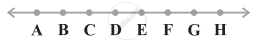Exercise 5.3 | Q 4 | Page 50

In the figure, we have AB = BC, BX = BY. Show that AX = CY.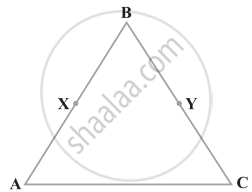Exercise 5.3 | Q 5 | Page 50

In the figure, we have X and Y are the mid-points of AC and BC and AX = CY. Show that AC = BC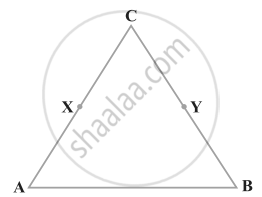Exercise 5.3 | Q 6 | Page 50

In the figure, we have BX = 1/2 AB, BY = 1/2 BC and AB = BC. Show that BX = BY.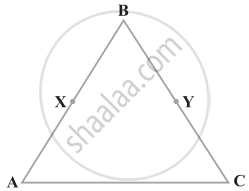Exercise 5.3 | Q 7 | Page 51

In the figure, we have ∠1 = ∠2, ∠2 = ∠3. show that ∠1 = ∠3.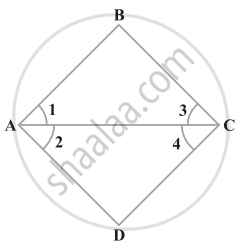Exercise 5.3 | Q 8 | Page 51

In the figure, we have ∠1 = ∠3 and ∠2 = ∠4. show that ∠A = ∠C.Exercise 5.3 | Q 9 | Page 51

In the figure, we have ∠ABC = ∠ACB, ∠3 = ∠4. Show that ∠1 = ∠2.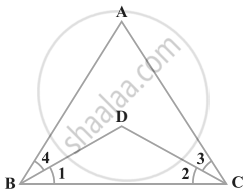Exercise 5.3 | Q 10 | Page 51

In the figure, we have AC = DC, CB = CE. Show that AB = DE.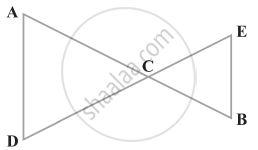Exercise 5.3 | Q 11 | Page 51

In the figure, if OX = 1/2 XY, PX = 1/2 XZ and OX = PX, show that XY = XZ.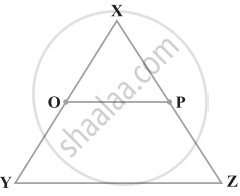Exercise 5.3 | Q 12.(i) | Page 52

In the figure AB = BC, M is the mid-point of AB and N is the mid-point of BC. Show that AM = NC.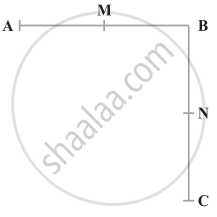Exercise 5.3 | Q 12.(ii) | Page 52

In the figure BM = BN, M is the mid-point of AB and N is the mid-point of BC. Show that AB = BC.Exercise 5.4 [Pages 52 - 53]

### NCERT solutions for Mathematics Exemplar Class 9 Chapter 5 Introduction To Euclid's Geometry Exercise 5.4 [Pages 52 - 53]

Exercise 5.4 | Q 1 | Page 52

An equilateral triangle is a polygon made up of three line segments out of which two line segments are equal to the third one and all its angles are 60° each.
Define the terms used in this definition which you feel necessary. Are there any undefined terms in this? Can you justify that all sides and all angles are equal in a equilateral triangle.

Exercise 5.4 | Q 2 | Page 53

Study the following statement:

“Two intersecting lines cannot be perpendicular to the same line”. Check whether it is an equivalent version to the Euclid’s fifth postulate.

Exercise 5.4 | Q 3 | Page 53

Read the following statements which are taken as axioms:

(i) If a transversal intersects two parallel lines, then corresponding angles are not necessarily equal.
(ii) If a transversal intersect two parallel lines, then alternate interior angles are equal.

Exercise 5.4 | Q 4 | Page 53

Read the following two statements which are taken as axioms:

(i) If two lines intersect each other, then the vertically opposite angles are not equal.
(ii) If a ray stands on a line, then the sum of two adjacent angles so formed is equal to 180°.

Exercise 5.4 | Q 5 | Page 53

(i) Things which are equal to the same thing are equal to one another.
(ii) If equals are added to equals, the wholes are equal.
(iii) Things which are double of the same thing are equal to one another.
Check whether the given system of axioms is consistent or inconsistent.

## Chapter 5: Introduction To Euclid's Geometry

Exercise 5.1Exercise 5.2Exercise 5.3Exercise 5.4## NCERT solutions for Mathematics Exemplar Class 9 chapter 5 - Introduction To Euclid's Geometry

NCERT solutions for Mathematics Exemplar Class 9 chapter 5 (Introduction To Euclid's Geometry) include all questions with solution and detail explanation. This will clear students doubts about any question and improve application skills while preparing for board exams. The detailed, step-by-step solutions will help you understand the concepts better and clear your confusions, if any. Shaalaa.com has the CBSE Mathematics Exemplar Class 9 solutions in a manner that help students grasp basic concepts better and faster.

Further, we at Shaalaa.com provide such solutions so that students can prepare for written exams. NCERT textbook solutions can be a core help for self-study and acts as a perfect self-help guidance for students.

Concepts covered in Mathematics Exemplar Class 9 chapter 5 Introduction To Euclid's Geometry are Concept for Euclid’S Geometry, Euclid’S Definitions, Axioms and Postulates, Equivalent Versions of Euclid’S Fifth Postulate.

Using NCERT Class 9 solutions Introduction To Euclid's Geometry exercise by students are an easy way to prepare for the exams, as they involve solutions arranged chapter-wise also page wise. The questions involved in NCERT Solutions are important questions that can be asked in the final exam. Maximum students of CBSE Class 9 prefer NCERT Textbook Solutions to score more in exam.

Get the free view of chapter 5 Introduction To Euclid's Geometry Class 9 extra questions for Mathematics Exemplar Class 9 and can use Shaalaa.com to keep it handy for your exam preparation